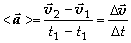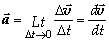# Physics Assignment Help With Average Acceleration and Instantaneous Acceleration## Average Acceleration and Instantaneous Acceleration

Very often, the velocity of a particle changes either in magnitude, in direction, or both as the motion proceeds. The body is then said to have acceleration. The acceleration is defined as the time rate of change of velocity. The average acceleration is defined asThe direction of average acceleration (vector) is the direction of change in velocity (vector). Its unit is m/s2.

The instantaneous acceleration is defined byIn general, there is no relation between the direction of velocity vector and the direction of acceleration vector.

### Assignment Help For Average Acceleration and Instantaneous Acceleration

assignmenthelp.net provides best Online Assignment Help service in Physics for all standards. Our Tutor provide their high quality and optimized Tutorial help to fulfill all kind of need of Students.